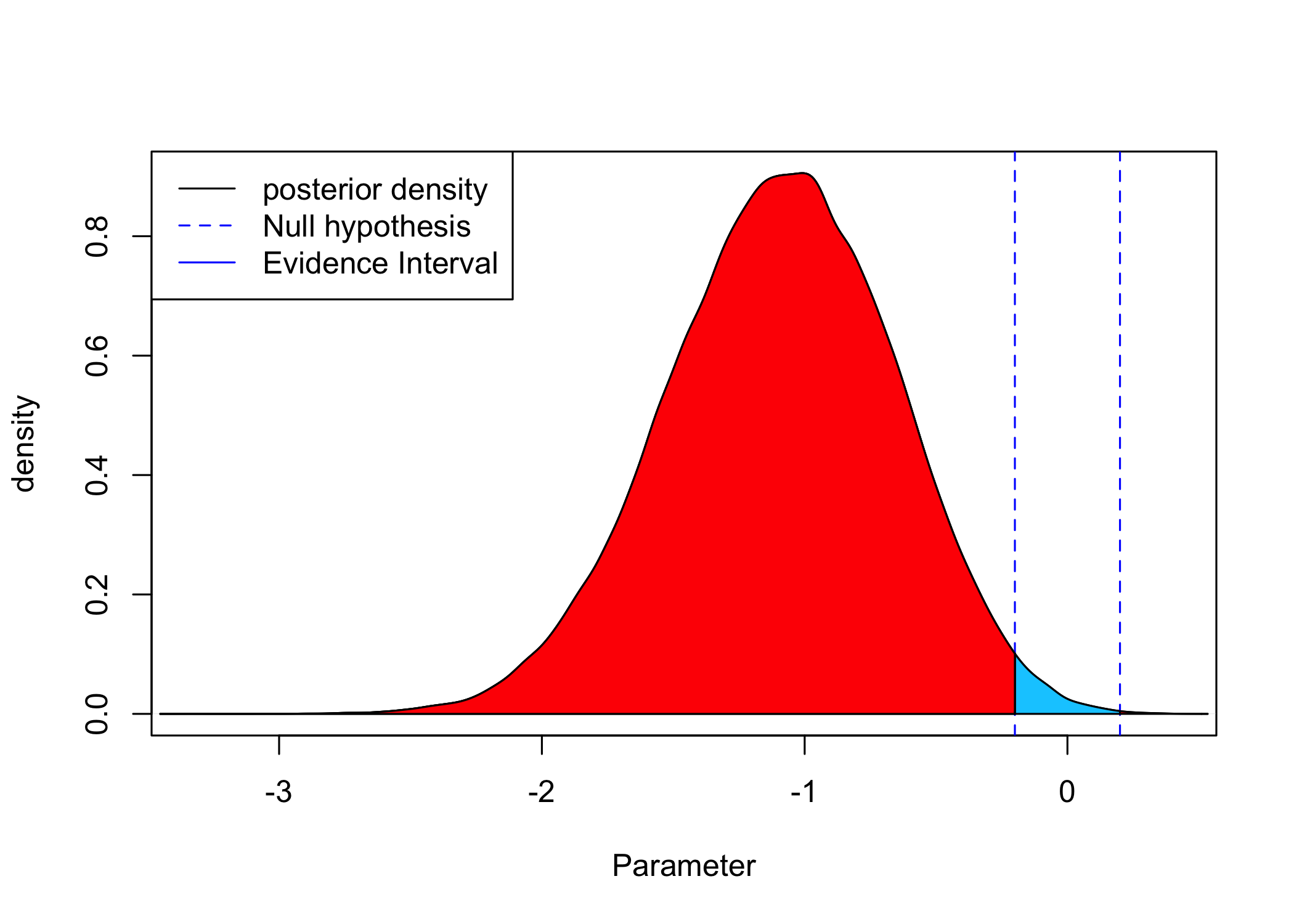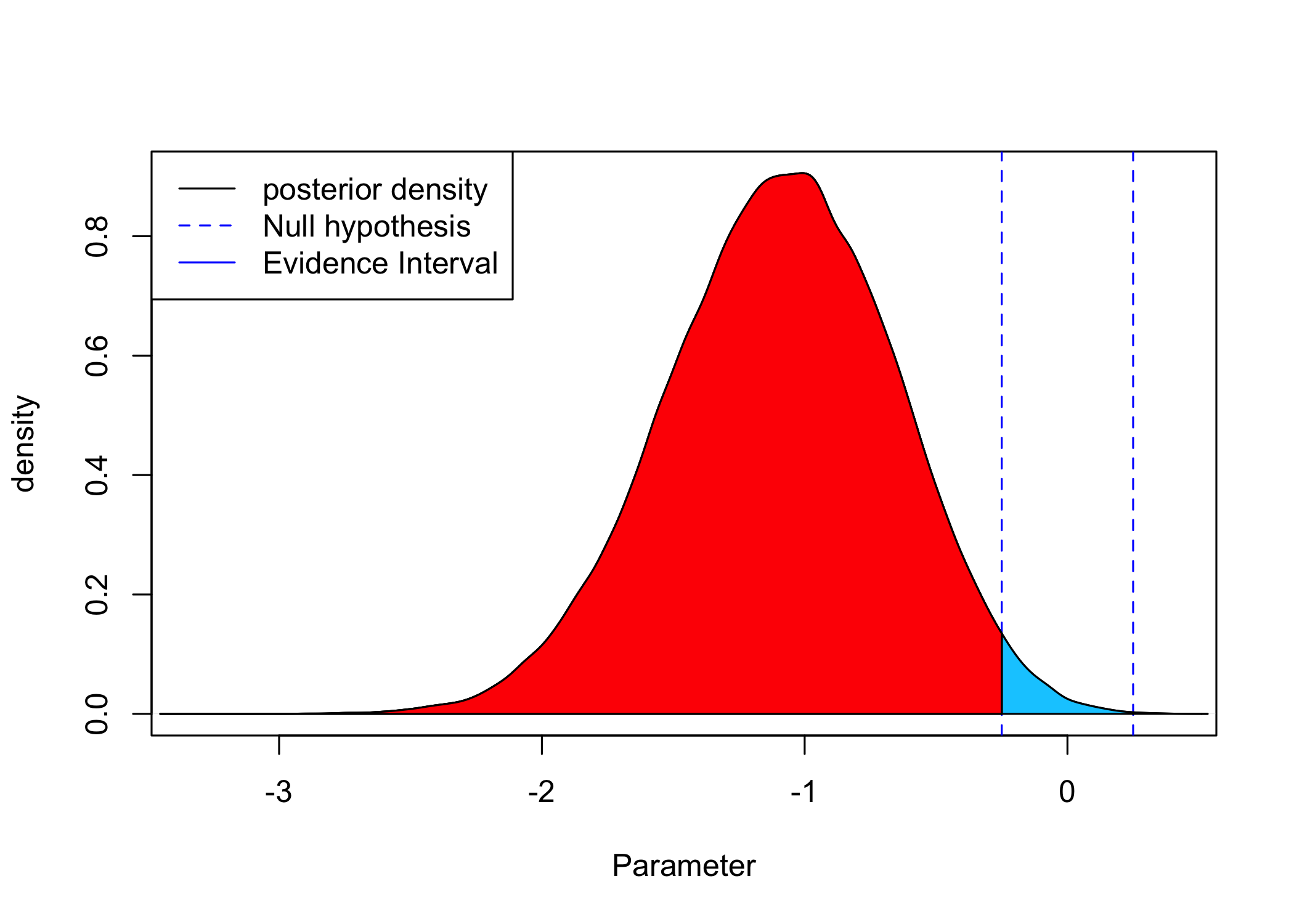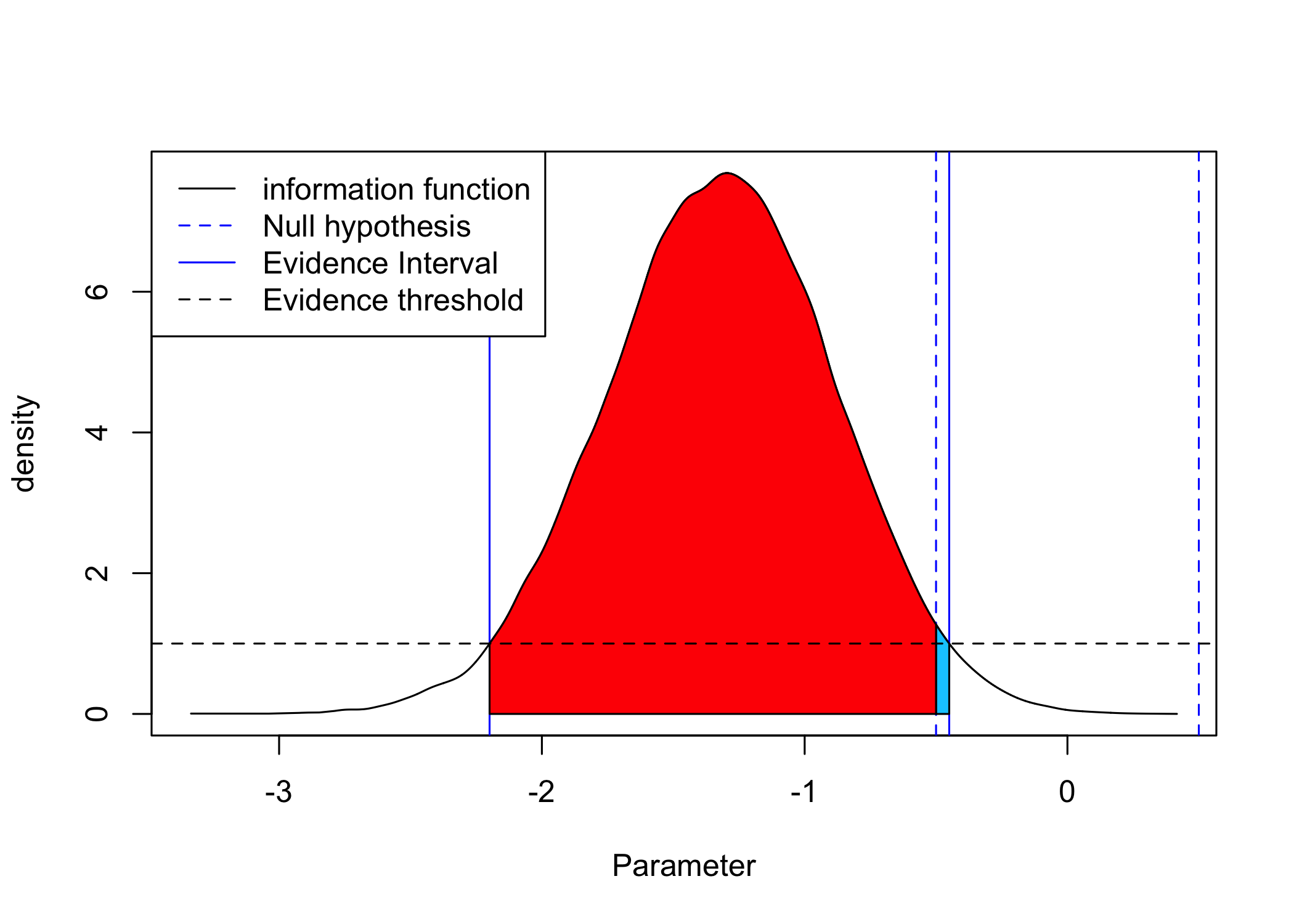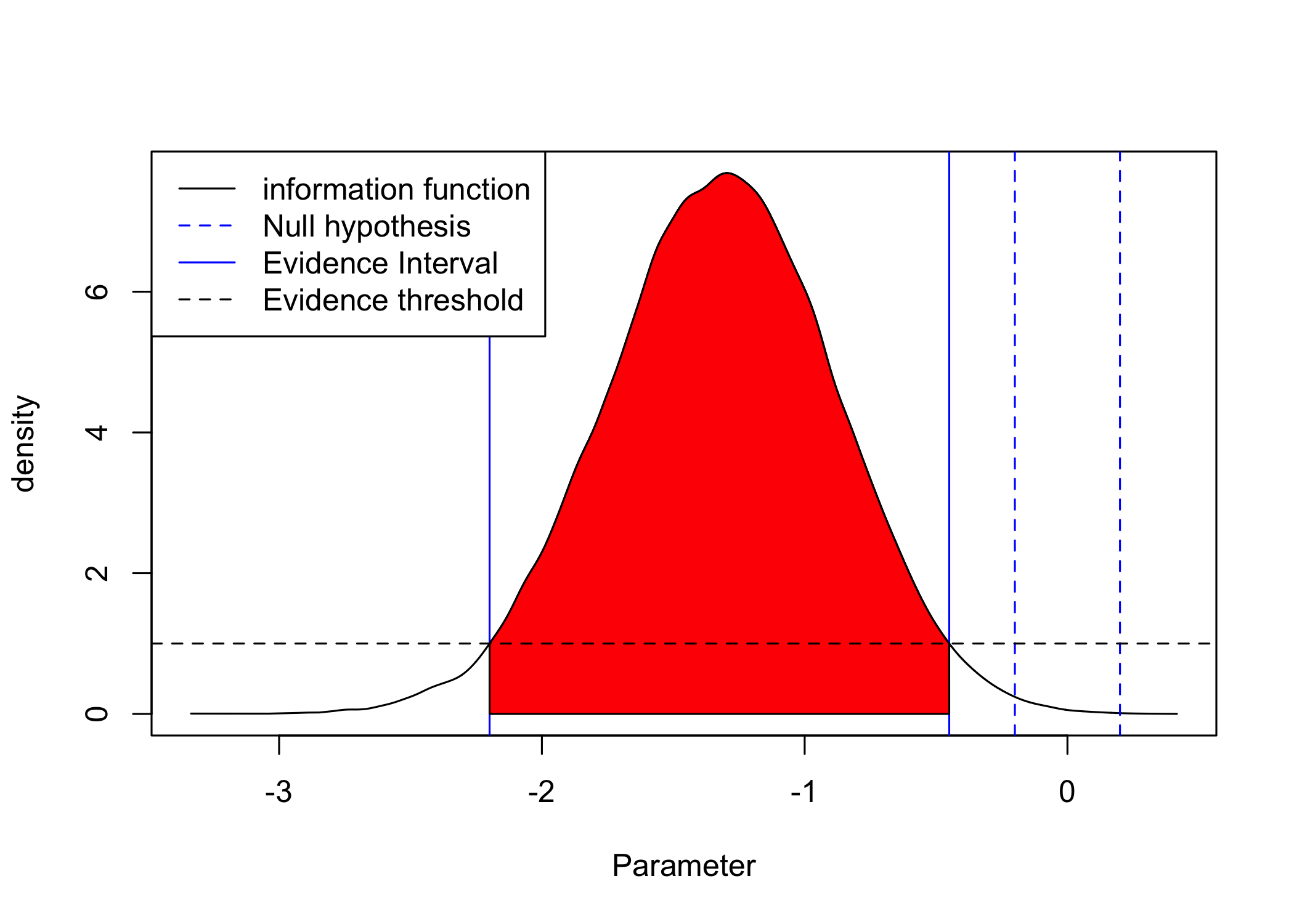# Bayesian hypothesis testing with the Full Bayesian Evidence Test

## Bayesian hypothesis testing with the Full Bayesian Evidence Test

Author: Riko Kelter

library(fbst)
#> This is rstanarm version 2.21.3
#> - See https://mc-stan.org/rstanarm/articles/priors for changes to default priors!
#> - Default priors may change, so it's safest to specify priors, even if equivalent to the defaults.
#> - For execution on a local, multicore CPU with excess RAM we recommend calling
#>   options(mc.cores = parallel::detectCores())

This vignette explains how to use the Full Bayesian Evidence Test (FBET) for Bayesian hypothesis testing of an interval hypothesis against its alternative via the Bayesian evidence value, which includes the e-value of the FBST as a special (limiting) case.

## Theory behind the FBET and Bayesian evidence value

While the Full Bayesian Significance Test (FBST) uses the e-value to quantify the evidence against a precise hypothesis, in a variety of contexts the assumption of a precise point-null hypothesis is unrealistic. Often, for example in biomedical research or the cognitive sciences, the assumption of an interval hypothesis is more appropriate. The Full Bayesian Evidence Test (FBET) generalizes the Pereira-Stern-theory which underpins the FBST and allows for testing interval hypotheses. The FBET can be used with any standard parametric model, where $$\theta \in \Theta \subseteq \mathbb{R}^p$$ is a (possibly vector-valued) parameter of interest, $$p(x|\theta)$$ is the likelihood and $$p(\theta)$$ is the density of the prior distribution $$\mathbb{P}_{\vartheta}$$ for the parameter $$\theta$$.

### The Bayesian evidence interval

First, the Bayesian evidence interval is required for the FBET. Let $$s(\theta):=\frac{p(\theta|y)}{r(\theta)}$$ be the surprise function of the Pereira-Stern FBST with a given reference function $$r(\theta)$$ and tangential set $$\overline{T}(\nu):=\{\theta \in \Theta|s(\theta)> \nu \}$$ to the null hypothesis $$H_0:\theta=\theta_0$$. The expanded tangential set is defined as $$\tilde{\overline{T}}(\nu)=\{\theta \in \Theta|s(\theta)\geq \nu \}$$, which in contrast to $$\overline{T}(\nu)$$ also includes the parameter values $$\theta$$ for which $$s(\theta)= \nu$$.

The Bayesian evidence interval $$\text{EI}_r(\nu)$$ with reference function $$r(\theta)$$ to level $$\nu$$ includes all parameter values $$\theta$$ for which $$\theta \in \tilde{\overline{T}}(\nu)$$.

It can be shown that the Bayesian evidence interval includes standard HPD intervals, support intervals and credible intervals as special cases.

To unify Bayesian interval estimation and hypothesis testing based on the $$\text{EI}_{r}(\nu)$$ via an interval hypothesis (or the region of practical equivalence (ROPE)), the Bayesian evidence value is introduced, which is associated with a Bayesian evidence interval: For a given evidence interval $$\text{EI}_r(\nu)$$ with reference function $$r(\theta)$$ to level $$\nu$$, the Bayesian evidence value $$\text{Ev}_{\text{EI}_r(\nu)}^{R}(H_0)$$ to a ROPE $$R$$ for the null hypothesis $$H_0$$ is given as $\text{Ev}_{\text{EI}_r(\nu)}^{R}(H_0):=\int_{\text{EI}_r(\nu) \cap R} p(\theta|y)d\theta$ The corresponding Bayesian evidence value $$\text{Ev}_{\text{EI}_r(\nu)}^{R}(H_1)$$ to a ROPE $$R$$ for the alternative hypothesis $$H_1$$ is given as $\text{Ev}_{\text{EI}_r(\nu)}^{R}(H_1):=\int_{\text{EI}_r(\nu) \cap R^c} p(\theta|y)d\theta$ where $$R^c$$ is the set complement of the ROPE $$R$$. The Bayesian evidence value $$\text{Ev}_{\text{EI}_r(\nu)}^{R}(H_0)$$ is the integral of the posterior density $$p(\theta|y)$$ over the intersection of the evidence interval $$\text{EI}_r(\nu)$$ with the ROPE (or interval hypothesis) $$R$$. This means that the evidence value $$\text{Ev}_{\text{EI}_r(\nu)}^{R}(H_0)$$ is the integral over the evidence interval $$\text{EI}_r(\nu)$$ restricted to the ROPE (or interval hypothesis) $$R$$ around the null value of $$H_0:\theta=\theta_0$$. The Bayesian evidence value can also be called generalised e-value, as it can be shown to recover the e-value of the FBST as a special case.

The Bayesian evidence value depends on three quantities:

• The ROPE $$R:=[a,b], a<b$$, which includes the null value $$\theta_0$$ of $$H_0:\theta=\theta_0$$, that is $$\theta_0 \in R$$. Thus, the ROPE $$R$$ can be interpreted as an interval hypothesis $$H_0:=[a,b]$$ around the null value $$\theta_0$$.
• the reference function $$r(\theta)$$ which is used for construction of the Bayesian evidence interval $$\text{EI}_r(\nu)$$
• and the evidence-threshold $$\nu$$ used for deciding which values are included in the Bayesian evidence interval $$\text{EI}_r(\nu)$$

The evidence value of course also depends on the prior density $$p(\theta)$$, because the Bayesian evidence interval depends on the posterior density, which itself depends on the prior density $$p(\theta)$$. Of course, the Bayesian evidence value also depends on the data $$y$$ observed.

From a hypothesis testing perspective a ROPE $$R$$ is interpreted as an interval hypothesis which includes the precise null value of the hypothesis $$H_0$$. Thus, from this point of view one could also omit the superscript $$R$$ in $$\text{Ev}_{\text{EI}_r(\nu)}^{R}(H_0)$$ and define the Bayesian evidence value directly for interval hypotheses $$H_0 \in \Theta$$ as $\text{Ev}_{\text{EI}_r(\nu)}(H_0):=\int_{\text{EI}_r(\nu) \cap H_0} p(\theta|y)d\theta$ The general approach to consider a (small) interval hypothesis instead of a point-null hypothesis goes back to Hodges & Lehmann (1954).

## Running the FBET

To run the FBET, a posterior distribution is required, which can be obtained as in any standard Bayesian workflow:

1. Specify a joint distribution for the outcome(s) and all relevant parameters. Typically, this takes the form of a marginal prior distribution for the parameters multiplied by a likelihood for the outcome(s) conditional on the parameters. This joint distribution is proportional to a posterior distribution of the parameters conditional on the observed data.
2. Run a Markov-Chain-Monte-Carlo (MCMC) algorithm to draw samples from posterior distribution.
3. Evaluate the model fit with regard to the data (e.g. via posterior predictive checks) and possibly revise the model.

Based on the sample of posterior draws, the FBET can be performed as follows:

1. Specify a null hypothesis value $$\theta_0$$ for the precise null hypothesis $$H_0:\theta=\theta_0$$.
2. Specify a ROPE $$R$$ around the null hypothesis value $$\theta_0$$ based on prior subject-domain knowledge, pilot studies or by deciding for minimally relevant effect sizes that can be regarded as scientifically meaningful.

Conceptually, steps one and two can also be merged into a single step which specifies an interval hypothesis $$H_0:\theta \in [\theta_0-\varepsilon_1,\theta_0+\varepsilon_2]$$ around $$\theta_0$$ for $$\varepsilon_1,\varepsilon_2>0$$.

1. Specify a reference function $$r(\theta)$$ which is used to build the surprise function $s(\theta):=\frac{p(\theta|y)}{r(\theta)}$ Typical choices include a flat reference function $$r(\theta):=1$$ or the density $$p(\theta)$$ of a prior distribution $$P_{\vartheta}$$ for the parameter $$\theta$$. In the former case, the surprise function becomes the posterior density $$p(\theta|y)$$. In the latter case, the surprise function $$s(\theta)$$ quantifies the ratio of posterior to prior, and parameter values $$\theta$$ with $$s(\theta)\geq 1$$ can be interpreted as being corroborated by observing the data $$y$$.

As a sidenote, the connection between statistical surprise, corroboration and statistical information is non-trivial and the interested reader is referred to Good (1968) for a detailed treatment of the topic. However, for current purposes it is legitimate to speak of corroboration in the above context, although being corroborated could be replaced by being informative with regard to $$H_0$$ would be more accurate. Parameter values $$\theta$$ with $$s(\theta)<1$$ can be interpreted as not being corroborated by observing the data $$y$$.

1. Select the evidence-threshold $$\nu \geq 0$$ and calculate the resulting Bayesian evidence interval $$\text{EI}_r(\nu)$$ for the reference function $$r(\theta)$$ and threshold $$\nu$$. The Bayesian evidence interval $$\text{EI}_r(\nu)$$ includes only parameter values $$\theta \in \Theta$$ which have been corroborated by a factor $$\nu$$ compared to the reference function $$r(\theta)$$, that is, parameter values $$\theta$$ for which the evidence is at least $$\nu$$.
2. Run the FBET and compute the Bayesian evidence value $$\text{Ev}_{\text{EI}_r(\nu)}^{R}(H_0)$$ in favour of $$H_0$$, and the Bayesian evidence value $$\text{Ev}_{\text{EI}_r(\nu)}^{R}(H_1)$$ in favour of $$H_1$$.

Note that the notion of evidence associated with the Bayesian evidence interval $$\text{EI}_r(\nu)$$ stems from the relationship to the Bayes factor: Parameter values $$\tilde{\theta} \in \text{EI}_r(\nu)$$ fulfill the condition $$s(\tilde{\theta})\geq \nu$$, which is equivalent to $$p(\tilde{\theta}|y)/r(\tilde{\theta})\geq \nu$$. Whenever the reference function $$r(\theta)$$ is chosen as the model’s prior distribution $$p(\theta)$$ for the parameter $$\theta$$, for the values $$\tilde{\theta}$$ the ratio $$p(\theta|y)/p(\theta)$$ can be identified as the Savage-Dickey-density ratio which is equal to the Bayes factor $$BF_{01}$$ for the precise null hypothesis $$H_0:\theta=\tilde{\theta}$$ when Dickey’s continuity condition holds. Thus, the evidence for $$H_0:\theta=\tilde{\theta}$$ obtained through a traditional Bayes factor hypothesis test is at least $$\nu$$ for all values inside the Bayesian evidence interval $$\text{EI}_r(\nu)$$.

Again, as a sidenote it is worth mentioning that the Bayes factor is simply a difference in weak explanatory power, where the latter is equal to statistical information, where again the latter term is boroughed from information theory and, in particular, Kullback-Leibler information (or divergence). Thus, the evidence interval has close connections to information theory and also to weight of evidence, see Good (1968).

Now, we illustrate the FBST with two examples below. The first example is a Bayesian two-sample t-test with flat reference function, and the second example the same test with a user-defined reference function.

## Examples

### Example 1: Paired-sample t-test with flat reference function

We use Student’s sleep data as illustration, which is available in the data frame sleep.

sleep
#>    extra group ID
#> 1    0.7     1  1
#> 2   -1.6     1  2
#> 3   -0.2     1  3
#> 4   -1.2     1  4
#> 5   -0.1     1  5
#> 6    3.4     1  6
#> 7    3.7     1  7
#> 8    0.8     1  8
#> 9    0.0     1  9
#> 10   2.0     1 10
#> 11   1.9     2  1
#> 12   0.8     2  2
#> 13   1.1     2  3
#> 14   0.1     2  4
#> 15  -0.1     2  5
#> 16   4.4     2  6
#> 17   5.5     2  7
#> 18   1.6     2  8
#> 19   4.6     2  9
#> 20   3.4     2 10

We select both groups and perform a traditional Bayes factor paired-sample t-test based on the model of Rouder et al. (2009). There, the null hypothesis states that the standardized effect size $$\delta:=(\mu-\mu_0)/\sigma$$ is assumed to be zero under $$H_0$$, and Cauchy-distributed under the alternative $$H_1$$, $$\delta \sim C(0,\gamma)$$ for $$\gamma>0$$.

require(BayesFactor)
#> ************
#>
#> Type BFManual() to open the manual.
#> ************
#>
#> Attache Paket: 'BayesFactor'
#> Das folgende Objekt ist maskiert 'package:ks':
#>
#>     compare
grp1 = sleep[1:10,]$extra grp2 = sleep[11:20,]$extra
diff = grp1 - grp2
ttestBF(x = grp1, y = grp2, rscale = "medium", paired = TRUE)
#> Bayes factor analysis
#> --------------
#>  Alt., r=0.707 : 17.25888 ±0%
#>
#> Against denominator:
#>   Null, mu = 0
#> ---
#> Bayes factor type: BFoneSample, JZS

Based on a traditional Bayes factor test, there is strong evidence for the alternative $$H_1:\delta \neq 0$$: $$BF_{10}=17.25$$ according to Jeffreys (1961). However, note that the precise Bayes factor tests the point-null hypothesis $$H_0:\delta=0$$ of exactly no effect, that is, no difference between both population means. Without observing any data $$y$$, we can be reasonable sure that the effect size $$\delta$$ will almost surely not be exactly zero, so an interval hypothesis which separates scientifically meaningful from scientifically irrelevant parameter values constitutes a more adequate test in such a situation (interval Bayes factors would be more adequate when sticking to Bayes factors).

Therefore, we now obtain posterior MCMC samples and conduct the FBET based on a flat reference function $$r(\theta):=1$$. We use the evidence threshold $$\nu=0$$, which implies that the surprise function $$s(\theta)=p(\theta|y)/r(\theta)$$ reduces to the posterior density $$p(\theta|y)$$ and the Bayesian evidence interval $$\text{EI}_r(\nu)$$ becomes $$\text{EI}_r(0)$$, which includes all parameter values $$\theta \in \Theta$$ with positive posterior density $$p(\theta|y)\geq 0$$.

set.seed(42)
posteriorDraws = ttestBF(x = grp1, y = grp2, rscale = "medium", paired = TRUE,
posterior = TRUE, iterations = 100000)[,3]
set.seed(42)
result = fbet(posteriorDensityDraws = posteriorDraws, interval = c(-0.2,0.2), nu=0)
summary(result)
#> Full Bayesian Evidence Test:
#> Reference function: Flat
#> Testing Hypothesis H_0:=[ -0.2 , 0.2 ]
#> Bayesian e-value in favour of H_0: 0.01418125
#> Bayesian e-value against H_0: 0.9858188

The Bayesian evidence value $$\text{Ev}_{\text{EI}_r(\nu)}^{R}(H_0)$$ in favour of $$H_0:\theta \in [-0.1,0.1]$$ is equal to $$0.014$$, while the Bayesian evidence value $$\text{Ev}_{\text{EI}_r(\nu)}^{R}(H_1)$$ in favour of $$H_1$$ is equal to $$0.986$$. Thus, the evidence for the alternative $$H_1:\theta \notin [-0.1,0.1]$$ is much stronger than the evidence in favour of $$H_0$$. In fact, $$98.6\%$$ of the posterior probability mass are located outside the interval $$[-0.1,0.1]$$, which is expressed by the Bayesian evidence value in favour of $$H_1$$. The following plot illustrates this result:

plot(result, type = "posterior")The blue area amounts to the $$0.5\%$$ of posterior probability mass which are located inside $$[-0.1,0.1]$$ and corroborate the null hypothesis $$H_0$$ based on the chosen ROPE $$R:=[-0.1,0.1]$$ around $$\theta_0:=0$$. However, the evidence threshold $$\nu=0$$ requires no form of corroboration for the parameter values $$\theta$$ after observing the data $$y$$, which is shown in the above plot: The evidence threshold is not visible, as it is a horizontal dashed line at the ordinate $$y=0$$.

If we choose a different hypothesis $$H_0:\delta \in (-0.25,0.25)$$, we obtain:

set.seed(42)
result2 = fbet(posteriorDensityDraws = posteriorDraws, interval = c(-0.25,0.25), nu=0)
summary(result2)
#> Full Bayesian Evidence Test:
#> Reference function: Flat
#> Testing Hypothesis H_0:=[ -0.25 , 0.25 ]
#> Bayesian e-value in favour of H_0: 0.02022616
#> Bayesian e-value against H_0: 0.9797738
plot(result2, type = "posterior")### Example 2: Paired-sample t-test with user-specified reference function

In the above, a flat reference function $$r(\theta):=1$$ was used in the surprise function $$s(\theta)$$ and the evidence threshold $$\nu$$ was set to zero. However, the prior distribution on the parameter $$\theta$$ was a medium Cauchy prior $$C(0,\sqrt{2}/2)$$. As the prior beliefs are reflected via this prior, it is reasonable to choose the Cauchy prior as the reference function instead (then, the surprise function is equal to what Good (1968) motivated as statistical information, providing an independent justification of the expanded tangential set; the latter includes parameter values which are informative with regard to the hypothesis under consideration). Below, it is shown how to do this. Also, the evidence threshold $$\nu$$ is set to $$\nu=1$$, so that only parameter values $$\theta$$ are included in the Bayesian evidence interval $$\text{EI}_r(1)$$ which attain a larger posterior density value than the corresponding prior density value. This time, we test $$H_0:\delta \in (-0.5,0.5)$$ against $$H_1:\delta \notin (-0.5,0.5)$$:

set.seed(42)
result3 = fbet(posteriorDensityDraws = posteriorDraws, interval = c(-0.5,0.5),
nu=1, FUN = dcauchy, par=list(location = 0, scale = sqrt(2)/2))
summary(result3)
#> Full Bayesian Evidence Test:
#> Reference function: User-defined
#> Testing Hypothesis H_0:=[ -0.5 , 0.5 ]
#> Bayesian e-value in favour of H_0: 0.01757639
#> Bayesian e-value against H_0: 0.9117274

Now, based on the different reference function and evidence threshold, we see that the resulting Bayesian evidence values change accordingly. Below, the situation is illustrated:

plot(result3, type="surprise")The horizontal dashed line shows the evidence-threshold $$\nu=1$$, and only parameter values $$\theta$$ which fulfill $$p(\theta|y)/p(\theta)\geq 1$$ are included in the Bayesian evidence interval $$\text{EI}_r(1)$$. The resulting Bayesian evidence interval $$\text{EI}_r(1)$$ is shown as the vertical blue lines in the plot. The interval hypothesis $$H_0:=(-0.5,0.5)$$ is shown as the dashed vertical blue lines, and the resulting Bayesian evidence value $$\text{Ev}_{\text{EI}_r(\nu)}^{R}(H_0)$$ in favour of $$H_0$$ is visualized as the blue area, which amounts to $$1.75\%$$ of the posterior probability mass. The Bayesian evidence value $$\text{Ev}_{\text{EI}_r(\nu)}^{R}(H_1)$$ in favour of $$H_1$$ is given as $$\text{Ev}_{\text{EI}_r(\nu)}^{R}(H_1)=0.9117$$, which shows that $$91.17\%$$ of the posterior probability mass confirm the alternative. It should be clear by now that omitting the ROPE $$R$$ and instead switching towards an interval hypothesis $$H_0$$ from the start only simplifies the notation. Henceforth, we therefore only speak of $$H_0$$.

Now, if we choose a different interval hypothesis $$H_0:=(-0.2,0.2)$$, we obtain:

result4 = fbet(posteriorDensityDraws = posteriorDraws, interval = c(-0.2,0.2),
nu=1, FUN = dcauchy, par=list(location = 0, scale = sqrt(2)/2))
summary(result4)
#> Full Bayesian Evidence Test:
#> Reference function: User-defined
#> Testing Hypothesis H_0:=[ -0.2 , 0.2 ]
#> Bayesian e-value in favour of H_0: 0
#> Bayesian e-value against H_0: 0.9293033

Now, based on the different reference function and evidence threshold, we see that the resulting Bayesian evidence values change accordingly: The evidence for the null hypothesis reduces, while the evidence for the alternative has increased. Below, the situation is illustrated:

plot(result4, type="surprise")Next to plotting the surprise function we can also plot the resulting posterior density and visualize the evidence values. Therefore, we just need to omit the type argument.

plot(result4)